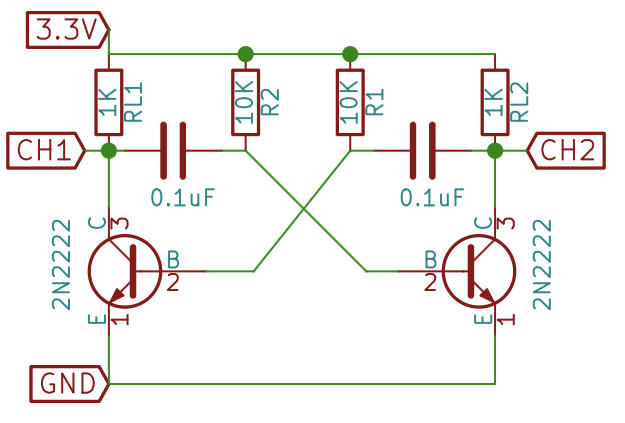## Performing Multivibrator Experiments in PSLab Android App

A Multivibrator is an Oscillator that produces non-sinusoidal signals like Square Wave. Multivibrators are considered to be the building blocks of almost every electronic device.

Multivibrators are the level changing circuit. Every circuit works on two level, “high” and “low”. Multivibrators changes between these two level to produce a particular voltage form.

helps us to observe the input and the output signals captured from these circuits. This enables student or researchers to study the input and output waveforms. Let’s discuss various Multivibrator Experiments that can be conducted using PSLab and how they are implemented.

There are three types of multivibrator:

1. Astable multivibrator
2. Bistable multivibrator
3. Monostable multivibrator

### Astable Multivibrator

An astable-multivibrator circuit’s output oscillates continuously between its two unstable states. It is a cross-coupled transistor switching circuit. They are also known as Free Multivibrator as any additional inputs or external assistance to oscillate are not required by them. Astable oscillators produce a continuous square wave from its output

Astable are used as clocks and timers, bistable as flip flops, the memory, registers and counters, Schmitt triggers as memory, switches, wave shapers.

The following is the circuit diagram.In order to observe the behaviour of Astable Multivibrator, LED’s can be also used.We get the following waveform when captured using the PSLab device.### Monostable Multivibrator

Monostable is also known as one shot multivibrator. In monostable multivibrator, there is one stable state and one astable state. A trigger pulse is required to enter into the astable state or get back to the stable state. The monostable multivibrator is mainly used as a timer.

The following is the schematics of Monostable MultivibratorFollowing signals are captured by the device while conducting the experiment.### Adding Multivibrator Experiment support in PSLab Android

This was simply achieved by reusing Oscilloscope Activity. Oscilloscope Activity is informed about the experiment by using putExtra() and getExtra() methods and Oscilloscope simply aligns its layout according to it.### Analysing Frequencies

In order to analyse the frequencies of the waves captured, we used sine fitting. Sine fitting function simply takes the data points and returns the amplitude, frequency, offset and phase shift of the wave.